# 8051 timer how to generate delay using 8051 timers

HOW TO USE TIMERS OF 8051 Microcontroller: 8051 microcontroller has two 16-bits built in timers. Each one of them can be individually programmed. Before learning about 8051 timer programming, you should know how to use keil for 8051 programming and how to use input put ports of 8051 microcontroller. These timers can either be configured as timers to generate a time delay or as counters to count events occurring outside the microcontroller. In this tutorial we will learn how to use Timers of 8051 microcontroller. The 8051 timers have three general functions:

1. Calculating the amounts of time between events.
2. Counting events.
3. Generating baud rate for serial port.

### Types of 8051 timers

8051 microcontroller has two timers: Timer0 and Timer1. Both of them can be used as timers or counters and both are 16-bits wide. But the architecture of 8051 is 8-bit, so each 16-bit is accessed as two separate registers of low byte and high byte.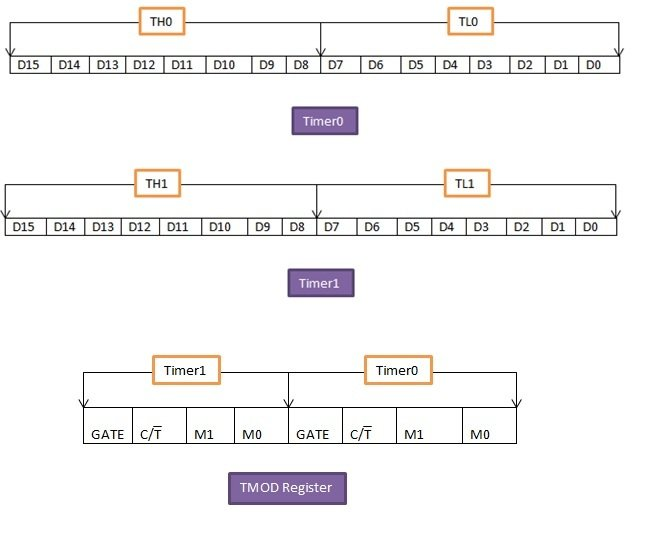8051 Timer0 Registers:
It is a 16-bit register and accessed as low byte and high byte. The low byte is referred as TL0 and the high byte is referred as TH0. These registers can be accessed like any other register.

8051 Timer1 Registers:
Timer1 is also a 16-bits register, which is split into two bytes, referred to as TL1 and TH1. Timer0 and timer1 share two common SFRs (special function registers): TMOD and TCON. We will discuss these two SFRs and see how they control timer0 and timer1.

### 8051 timer TMOD (timer mode) REGISTER

This timer is used to set various timer modes in timer0 and timer1. In this 8-bit TMOD register, 4 lower bits are set aside for timer0 and the 4 upper bits are used for timer1. For each timer, the lower 2 bits are used to set the timer mode while the remaining 2 upper bits are used to specify the operation.

GATE:           This bit is used for choice of internal or external control.

GATE=1, we can start and stop the timer from the external source
GATE=0, we don’t need external hardware to start and stop the timers

C/T:                This bit decides whether the timer is used as time delay generator or as an event counter.

C/T = 0, used as timer
C/T = 1,used as counter

MODE Bits M1, M0:

The last two bits for each timer i.e. M1 and M0 are used to select the timer mode. 8051 has 4 timer modes which are given below.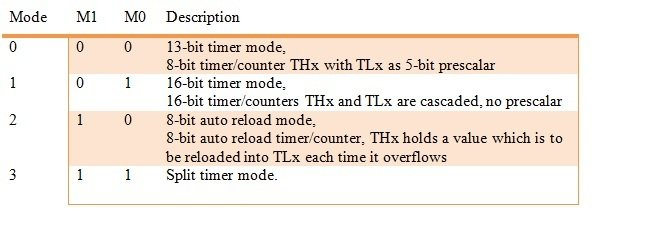Mode 1:

• It is a 16-bit timer.
• Values from 0000 to FFFFH can be loaded into thetimer’s registers TL and TH.
• Timer must be started after loading TH and TL with16-bit initial value.
• We can give initial values by using“SETB TR0” for timer0 and “SETB TR1” for timer1.
• When timer is started, it starts counting until it reaches its limit of FFFFH.
• After this limit of 0000H, it sets a TF (timer flag) bit, TF0 for timer0, TF0 and for timer1.
• Now stop the timer with the instructions “CLR TR0” for timer0 or “CLR TR1” for timer1.
• To repeat the process, the original values must be reloaded in TH and TL registers and timer flag must be reset to 0.

For time delay,the timer use the clock source of the crystal frequency of the crystal attached to 8051. The frequency for the timer will be 1/12th of the frequency of the external crystal attached.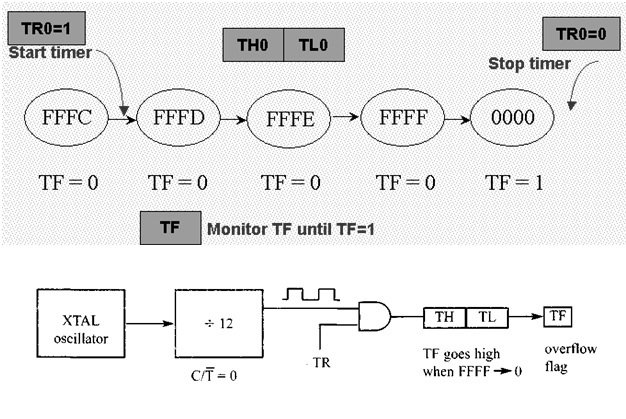Mode0:

• It is a 13-bit timer.
• It is same as mode 1 but with only difference of size.
• Mode0 register can hold values up to 1FFFH in TH-TL.
• When timer reaches its maximum of 1FFFH, it rolls over to 0000 and TF (flag bit) is raised.
• Not used generally.

Mode 2:

• It is an 8 bit timer.
• This timer first loads the values up to FFH in TH register.
• When TH is loaded, 8051 copies the same 8-bit value to TL.
• The timer must be started by ‘SETB TR0’ and ‘SETB TR1’ instructions for timer0 and timer1 respectively.
• When it starts, it continues to increment the TL register until it reaches FFH, after that it rolls over to 00 and raises the TF. After that TL is loaded automatically with its original value which is reserved by the register TH.
• The main feature of this mode is that it repeats the process.We just have to clear TF register and it will load original value to the register. While in mode1,programmer reloads the values to TH and TL.

Mode3:

• It is also known as a split timer mode.
• It splits timer0 in to two 8-bit timers.
• These 8 bit timers can count from 00H to FFH.
• This mode is used in the applications where we require an additional 8 bit timer or counter.
• TL0 sets TF0 and TH0 sets TF1.
• We can program timer0 and timer1 independently in mode 0, 1 and 2 of similar mode for other timer. But mode 3 can’t work independently.
• If we choose mode 3 for timer0 and we place timer1 in mode3 it will cause the timer to stop counting. Then timer 0 will use TR1 (timer1 register) and TF1 (timer1 flag) i.e. timer 0 has to depend on timer 1.

### 8051 timer TCON REGISTER

The second special function register is Timer control register. It is an 8 bit register and each bit has a special function. Bits, symbols and functions of every bits of TCON register are as follows: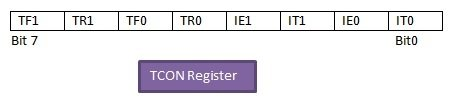TF1:    Over flow flag for Timer1.

TF1 = 1, Set when timer rolls from all 1s to 0
TF1 = 0, Cleared to execute interrupt service routine

TR1:   Run control bit for timer1.

TR1 =1 Timer1 Turn On
TR1 =0Timer1 Turn Off

TF0:    Over flow flag for timer0, same as TF1.

TR0:   Runcontrol bit for Timer0, same as TR1.

IE1:     External interrupt 1 Edge flag. It is not related to timer operations.

IT1:     External interrupt1 signal type control bit.

IT1 = 1, to enable external interrupt 1 to be triggered by a falling edge signal
IT1 = 0, to enable a low level signal on external interrupt 1 to generate an interrupt

IE0:     External interrupts 0 Edge flag. It is also not related to timer operations

IT0:     External interrupt 0 signal type control bit, same as IT1.

### DELAY CALCULATION of 8051 timer

Clock source:

Timer needs a clock source. If C/T = 0, the crystal frequency attached to the 8051 is the source of the clock for the timer. The value of crystal frequency attached to the microcontroller decides the speed at which the timer ticks. Now suppose that crystal frequency is 11.059MHz.

Timer’s clock frequency:

The frequency for the timer is always 1/12th of the frequency of the crystal attached to the 8051.

TF = 1/12  x 11.059MHz = 921583 Hz

Timer’s clock period:

The time delay of one machine cycle is given below. We use this to generate the delay.

TP = 1/ 921583= 1.085 µ sec

For delay of 10ms:

1. Firstly divide the desired time delay value (10 ms) by the timer clock period.

N=  1 / 1.085us

N= 10ms / 1.0859usec

N=9216

1. Subtract the value of N from the maximum number of counts possible for 16 bit timer i.e. 2^16 = 65536.

M=65536-N

M=65536-9216

M= 56320

1. Convert this value to hexadecimal and write in TH and TL registers.

MH=DC00H

TH=DCH

TL=00H

### 8051 timer generate delay

Take an example of creating a delay of 10ms using timers of 8051 microcontroller. Crystal used is of frequency 11.059MHz.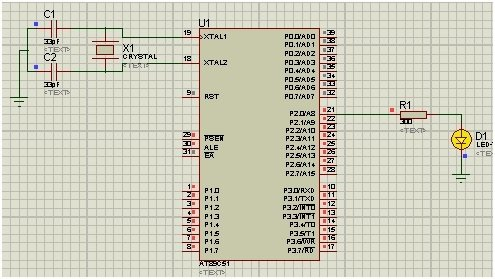### 8051 timer delay code

```#include<reg51.h>

sbit led=P2^0;                          // led at PORT 2 pin 0

void Delay(void);              // Delay function declaration

void main ()                        // main function

{

led=0;                                   //output PORT

while(1)                               // infinite loop

{

led = 1;                  // LED ON

Delay();

led = 0;                  // LED OFF

Delay();

}

}

void Delay()

{

TMOD = 0x01;           // Timer0 mode1

TH0=0xDC;              //initial value for 10ms

TL0=0x00;

TR0 = 1;               // timer0 start

while (TF0 == 0);      // check overflow condition

TR0 = 0;               // Stop Timer

TF0 = 0;               // Clear flag

}```

### 3 thoughts on “8051 timer how to generate delay using 8051 timers”

1. Thank you Sir.